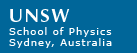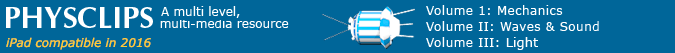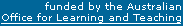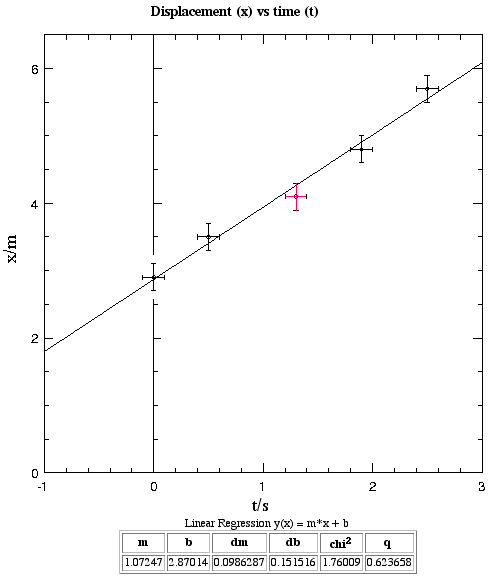# Graphs, errors, significant figures, dimensions and units图表,误差,有效数字,量度和单位

The first module in Physclips uses displacement-time and velocity-time graphs for a man walking in a straight line, so we'll begin with this animation.

An example: Displacement-time graphs (例子：位移时间图表)

How can we keep track of this fellow? In other words, how do we show his position at any time? The graph above answers this question. The man's distance from some reference position, here the wall, is how far he is displaced from it. We call this displacement x, where x is positive if he is to the right of the wall. Formally, the graph shows his position as a function of time.

The reference for displacement is the wall, x = 0. We also need a reference for time. It could be the time at which we set a stop-watch ticking. If the watch starts at zero seconds, any time after that is positive time (t > 0). Of course physics was still happening before we set our watch, so anything that happens before t = 0 would be represented on the negative part of the time axis.

Units on graphs (图表上的单位)

In science and engineering, the fundamental units for length and time are metres (abbreviation m) and seconds (s). Multiples and submultiples (kilometre, microsecond) are used when needed. There are two common ways of representing units on the axes of graphs (here m and s). One is to write x (m) and t (s). The disadvantage with this convention is that it may suggest that x is a function of m, and it is awkward when one really does want to plot x as a function of m.

The method used here is to plot x/m and t/s. This has the advantage that, when x is divided by a metre or t is divided by a second, the result is a number. Numbers (not quantities) are what we plot on the axes: the axes really are x/m and t/s, so it is a good idea to label them in this way.

 Errors, error bars and significant figures (误差，误差棒和有效数字)How accurately are the data known? Suppose you make the measurement x = 4.1 m by looking at a tape measure. As you do so, you click the stopwatch, which shows 1.32 seconds. It is difficult to define the position of a person with greater precision than about 0.2 m, because of the relative motion of arms and legs, so the error in the measurement is likely to be roughly this, or greater. We should therefore write x = 4.1 ± 0.2 m. 已知的数据有多精确? 假设你使用卷尺来测量x=4.1米。伴随着你测量距离, 你按下秒表, 时间记录1.32秒。很难去定义一个人的位置以0.2米的精确度, 由于手臂和腿的相对运动, 所以在测量中的误差可是大概和这个数值相等或者大于它。因此我们应当将位移写做x = 4.1 ± 0.2 m。 We might be tempted to write t = 1.32 s, because the watch is capable of measurements with this precision. You will probably find, however, that you are not. Repeated measurements of the same period (eg the time it takes a second hand to pass 2 seconds on a clock) will not usually give the same answer, and the variation is probably about 0.1 s. So the measurement error here is (very roughly) about 0.1 s. 你可能很想写下(t=1.32s), 因为秒表的精确度就是现实的数值. 但是你可以会发现并不是这样的. 重复测量相同的时间 (例如你用秒表测量一秒变到两秒的时间) 它通常不过有相同的结果, 变化可能是大概0.1秒。 所以这里测量的误差（粗略上将）是0.1秒. Now, if you write t = 1.32 s, you are implying that the '2' means something, that it is significant. You are implying a precision that you don't actually have. So, to avoid misleading the reader, you should retain only the two significant figures. Consequently, this should be written (as we say) to two significant figures, t = 1.3 s. To make the error explicit, we might write t = 1.3 ± 0.1 s. (If we had done a detailed study of our timing reproducibility and found its standard error to be 0.15 s, we could write t = 1.32 ± 0.15 s.) 如果你写t=1.32s, 你指的是最后一位'2'是有意义的, 这是有效位数. 你使用了一个你并没有实际拥有的有效位数. 所以为了避免误导读者, 你应当仅仅保留两位有效数字. 因此, 这个应该被写做两位有效数字（像我们说的那样), t = 1.3 s. 为了使误差更清楚, 我们可以写做 t = 1.3 ± 0.1 s. 如果我们通过完成详细的重复性的时间记录研究，发现它的标准误差是 0.15 s, 我们可以写做 t = 1.32 ± 0.15 s. How can we show the point (t,x) = (1.3 ± 0.1 s, 4.1 ± 0.2 m) on a graph? We do this by drawing a cross, whose vertical axis goes from 3.9 to 4.3 m and whose horizontal axis goes from 1.2 to 1.4 s. This is shown in red on the graph at right. 我们怎么能把点(t,x) = (1.3 ± 0.1 s, 4.1 ± 0.2 m)表现在图表上呢? 我们做了这样一个十字, 它的竖轴数值是从３.９到４.３米，横轴数值是从１.２秒到１.４秒. 这个显示在了右图的红色十字. The above is only a quick sketch. For more details, see the notes Errors and handling them. 以上的仅仅是快速简单的说明. 对于更详细的介绍, 可以参看资料误差和误差处理。 A good plotting program (好的绘图程序) Your computer probably came with spreadsheet software that draws graphs of the sort that administrative people like. That software is useful for storing the data and doing some manipulations, but the graphs it draws are unsuitable for science or engineering. 你的电脑可能具有电子制表软件，它可以根据用户的喜好分类绘图. 这些软件对于储存和管理数据是非常有用的, 但是它所画的图表并不适用于科学和工程学科. Michael Johnston, one of our past undergraduate physics students, was frustrated with the inability of such software to draw scientific graphs and to fit simple functions appropriately. So he wrote an application that does this. You'll note that it draws the error bars that you enter. If you ask to fit a simple function to the plot, it does a least-squares error-weighted fit: in other words, it gives more weight to the points with small errors than to those with large errors. Here is Michael's curve-fitting program., which we use to analyse an experiment in Projectiles and which we also used to make the graph at right. 一个前物理系的本科学生Michael Johnston, 对于这类软件在绘制科学图表以及简单公式拟合方面的缺陷表示非常失望. 所以他写了一个程序来执行这些任务. 你将会注意到它可以将你输入的误差棒绘制出来. 如果你需要绘制一个简单的拟合方程, 它能够做最小二乘法加权误差拟合: 换句话说, 它给了小误差点更多的权值相比于大误差点. 这里就是迈克尔的曲线拟合程序, 我们用它来分析抛物体实验中的数据，并且用它来绘制右方的图表.

Units and dimensions (单位和量度)

What is wrong with saying

- "My height is 45°C"? Or: 我的高度是４５摄氏度　或者
- "The game lasted 15 kg"? Or: 这场游戏持续了１５千克　或者
- "The displacement is given by the weight divided by the volume squared"?　位移定义为重量每平方体积

In each case, we mention two physical quantities that cannot possibly be equal. Let's take the first: a height cannot equal a temperature, so I cannot measure my height in degrees Celsius. Let's call my height h. I can say "h = 1.8 m". I can also say that h = V/A", where V is my volume and A is my average horizontal cross sectional area. In both cases, the quantity on the right hand side is a distance, as is the quantity on the left hand side. Both can be measured in metres.

We shall see that this very simple idea can be quite important -- and also very useful.

Now the units on either side of an equation need not be the same. For instance, I may write

1 inch = 25.4 mm.
1英寸=25.4毫米

This equation is true. (In fact, it is the definition of the inch, a unit of length in the old British system of units.) However, this equation is different from the silly examples given above, because both the millimetre and the inch measure length. We say that both sides of the equation have the dimensions of length. This condition must be satisfied for an equation to be true, or even to make sense. Further, if equations have different units with the same dimension, appropriate conversion factors must be included, as is the case above.

Let's look at more interesting examples. When we write

F  =  ma ,
we are specifying that the dimensions of force are those of mass times acceleration. The dimensions of acceleration are length, which we write as [L], divided by time [T] squared, so we write, just for the dimensions:

[F]  =  [M][L][T]−2.

In the units of the Système International, universally used in science, there are no conversion factors for the base units, so we can relate the newton, the unit of force, to other base units:

1 N  =  1 kg.m.s−2.
And to use our equation once more, we note that, while mass is a scalar, force and acceleration are both vectors, so our previous equation tells us not only that F = ma, but that F is parallel to a.

Let's now see how the method of dimensions can be useful, via this

Example: how does the frequency, of a pendulum depend on the length? (例子：一个钟摆的频率怎样取决于长度)

We know that this depends on the length, L -- a long one swings more slowly than does a short one. It also depends on the strength g of the gravitational field -- it won't swing at all without one. Does it also depend on the mass, m? On the temperature, T? Let's write for the frequency, f:

f  =  N.La.gb.mc.Td

where N, a, b, c and d are numbers, yet to be determined. Of course, we can analyse the dynamics of the pendulum and determine them, but let's see how far we get just by considering the dimensions. Frequency has units of "per second" so it has the dimensions of reciprocal time, T−1 . So, setting the dimensions equal on both sides, we have:

T−1  =  N.La.(L.T−2)b.Mc.Temperatured

For this equation to be true, each dimension must occur, to the same power, on each side. So, considering each dimension, the exponent gives us an equation to be satisfied. If we start with time T, we see that it appears to the −1 power on the left, and to the −2b power on the right, so we have

[T] ⇒   −1   =  −2b

[L] ⇒   0   =  a + b
[M] ⇒   0   =  c
[Temperature] ⇒   0   =  d.

Now the last two shouldn't surprise us. A more massive pendulum experiences a greater gravitational force but it also requires more force to accelerate, so we should not be surprised that the dimensions of the problem tell us that c = 0, ie that these effects cancel out: the frequency does not depend* on the mass of the pendulum. Similarly, we see that d = 0, but we should not be too surprised that a hot pendulum and a cold one swing at the same frequency -- unless of course the temperature changes the length perceptibly.

The other two equations tell us that b = 1/2, and that a + b = 0, so a = −a = −1/2. So, substituting in our original equation for the frequency,

f  =  N.L−1/2.g1/2  =  N(g/L)1/2.

We still don't know the value of the number N, and cannot get it from the information we have been given here. (It is 1/(2*π), in case you were wondering.) However, we do know that, all else equal, the frequency is proportional to the reciprocal of the square root of the length. To halve the frequency of pendulum, make it four times as long.

* I raise a couple of tiny caveats, to preempt the pedants. For a pendulum whose mass is comparable with the that of the planet upon which it is mounted, the pendulum mass does appear -- or at least the ratio of these two masses appears. Further, we have cheated a little on the temperature, because we could write temperature in units of energy. Doing so, the conversion factor would be Boltzmann's constant, whose very small size would give us the clue that temperature is only relevant in mechanics for objects of molecular size. And on this molecular scale we should often need to use quantum mechanics rather than Newtonian mechanics.
*  这里有几条附加说明, 用来应答一些学究. 对于一个重量可比拟于它所在的行星的重量的钟摆来说, 钟摆的重量M会出现在方程里-- 或者至少两个质量的比例会出现. 此外, 我们在温度方面的表示不完全正确, 因为我们可以把温度表现成能量). Doing so(这样做的话, 转换因子就是玻尔滋蔓常数, 这个非常小的常数向我们暗示着，仅仅当物体接近分子尺寸的时候温度才在机理中起作用. 在分子量级下，我们常常需要用量子力学而不是牛顿力学.

Other units (其他单位)

With rare exceptions, scientists use the SI system of units. (SI stands for Système International d'Unités.) This system is based on the kilogram for mass, the metre for length, the second for time, the ampere for electric current, the kelvin for temperature, the mole for chemical quantities and the candela for luminous intensity. Other systems are the British imperial system and natural units.

Physclips is a scientific presentation, and we use only the SI. If you encounter problems stated in other units, the simplest procedure is often to translate the problem into SI, solve it, then translate the back. This sounds like extra work, but it is usually much less than the extra work required in using the imperial system of units, which has internal conversion factors.
Physclips是一个科学介绍, 我们仅仅使用SI国际单位. 如果你在陈述其他单位的时候遇到了问题, 那么最简单的方法常常是把这个问题变成国际单位, 解决它, 然后再把单位变回来. 这听起来像是额外的工作, 但是这通常是比继续使用英制单位需要更少的额外工作, 英制单位具有内部转换因子.

In the United States of America, Liberia and Myanmar, the British imperial system is the official system. This system used to be much more widespread, and vestiges of it remain in other countries that are in the process of 'going metric', ie converting to the SI.

Dealing with or converting from the imperial system usually involves just a multiplicative factor. For instance, the inch, an imperial unit of length, is officially defined to be equal to 25.4 mm. These multiplications can become awkward in some cases: consider this imperial unit of thermal conductivity, one British Thermal Unit per second per square foot per degree Fahrenheit per inch. One can see why it exists, but it is ugly and inconvenient. (For comparison, the SI unit thermal conductivity is W.m-1.K-1.)

Some confusion arises, however, because of the different colloquial use of units of mass and force in the SI and imperial system. In the imperial system, the unit of force is the pound-force, or sometimes, as in many American physics text books, as just the pound. The unit of mass in the imperial system is the slug, which is a mass that is accelerated by one pound at one foot per second per second. The slug is 14.5939 kg. These equations, which are definitions, allow us to compare the units of mass and force:

SI(国际单位)

Unit of force(力的单位)  =  1 newton  =  1 kg.m.s−2
Imperial(英制单位)
Unit of force(力的单位)  =  1 pound  =  1 slug.foot.second−2

In imperial units, the gravitational acceleration is 32 feet.second−2. Consequently, a slug weighs 32 pounds.

The slug is very rarely used. Pound is used colloquially as a unit of quantity -- a pound of apples colloquially means a quantity of apples that weighs a pound-force (at the earth's surface). There is another imperial unit of force, the poundal. This is defined as the force required to accelerate at one foot.second−2 a mass whose weight is one pound. So a pound is 32 poundals.

The units mentioned above are related to features of the earth (its circumference originally determined the metre, and the second is related to the day) or of artifacts on earth, such as the standard kilogram, or of particular substances, especially water. The laws of physics and combinations of them yield natural units, which are used by some theoretical physicists, especially cosmologists. The speed of light, for instance, is taken as the unit for speed. Although this makes equations look simple these units are, in general, inconvenient for measurement. For instance, the natural units of length and time are inconveniently small (The Planck length is 1.6 x 10-35 metres, the Planck time is 5.4 x 10-44 seconds). See The Planck scale for more detail.This work is licensed under a Creative Commons License.